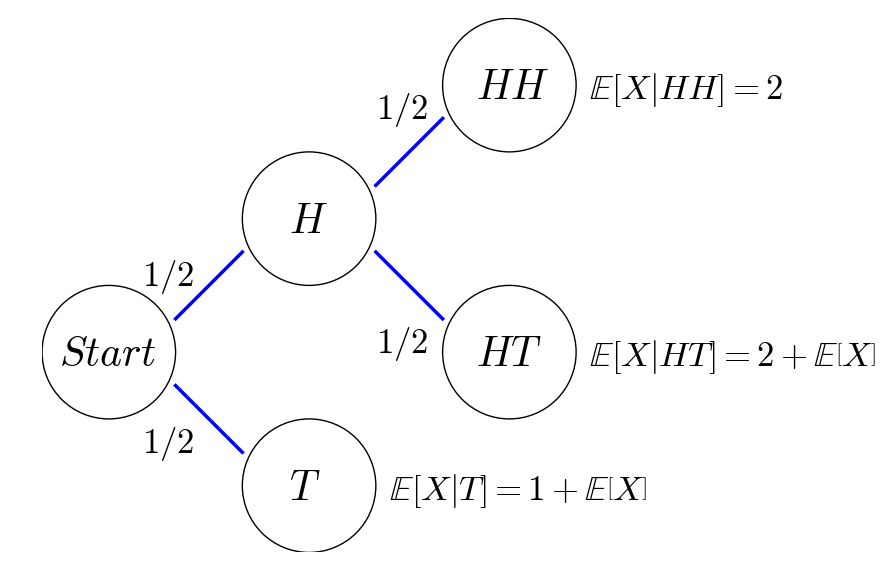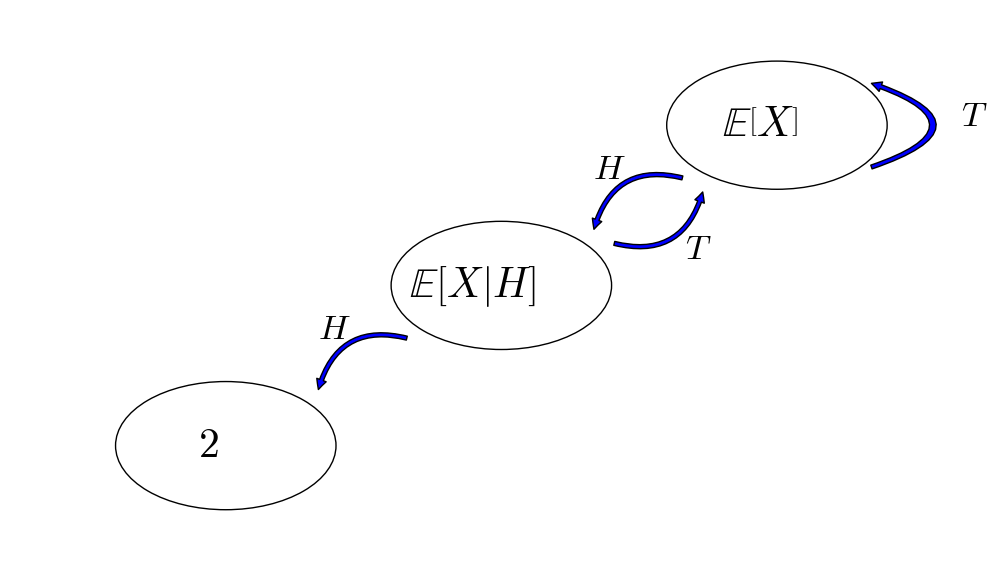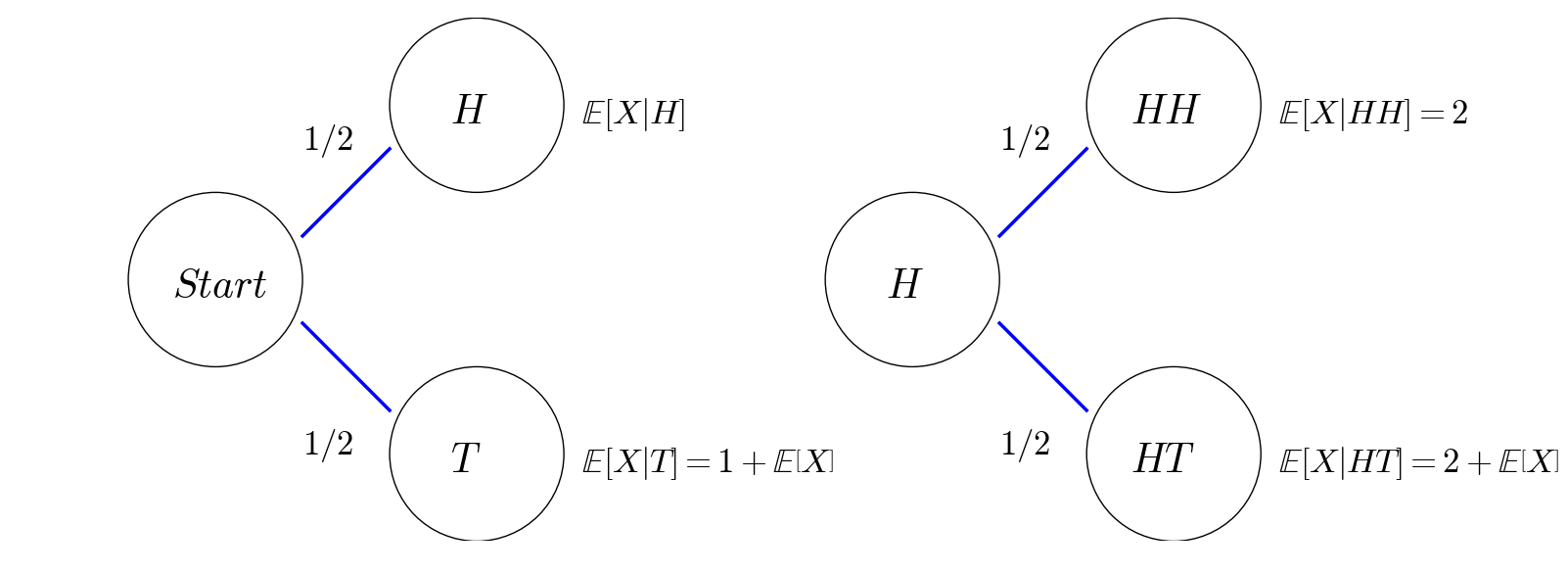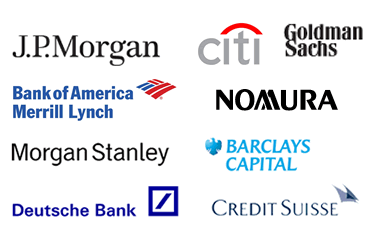# Reservoir Sampling

How to randomly and uniformly smaple k items out of a population (of an unknow size)?

# More On Expectations

## Problem 1Source

## Problem

Suppose we toss a fair coin, what is the expected number of tosses until we get two heads in a row?

I’ll show three ways of solving this problem.

## Method I: Recursive Expectation in Two Steps

Let $X$ be the number of tosses until we get two heads in a row, then we use the recursive relations of expectations to write down an equation of $\mathbb{E}\left[X\right]$ as follows:

This recursive relation can be better explained by the probabilistic tree below:For those who know Markov chains, we can also draw a state transition diagram as follows:## Method II: Recursive Expectation in One Step

This mehod is almost the same as Method I, except that we use the recursive relations of expections to write down a system of equations to solve:As you see more examples in my next post, it is more common to formulate a system of equation, rather than a single equation, for the problem of recursive expectations.

## Method III: Martingale Approach (Optional)

We introduce a random process $X_n,n\geq 1$ as a random process to represent the coin toss process, that is,

Note that $X_n$ is a martingale. And we use another random process $Y_n,n\geq 1$ to represent our trading strategy,

This means that we start our first bet with $1$, then we double our bet to $2$ if the previous coit toss turns out to be head and still bet $1$ otherwise.

Consequently, our wealth process $Z_n = Y_1X_1 + \sum_{i=2}^n Y_i(X_i - X_{i-1})$ is given by

Indeed, $Z_n$ is a discrete-time stochastic integral with respect to $X_n$, and therefore, $Z_n$ is also a martingale.

Now, we define a stopping time $\tau = \min\{n\geq 2: X_n = 1, X_{n-1}=1\}$. The definition of $\tau$ would imply that $X_1=\cdots=X_{\tau-2}=0,X_{\tau-1}=X_{\tau}=1$.

Finally, we invoke the optinal sampling theorem to get

# How to Get a Financial Quant Job? // 如何找到一份金融宽客的工作？

In this post, I will share some resources and practical tips about how to get a quant job.

## PreparationSource

Generally, a quant needs to know:

• Programming
• Math
• Stats (Machine Learning)
• Finance

Note: math used to be importmant when derivative pricing was just becoming popular, however, nowdays I feel that the demand for quants who build mathematical models decreases dramatically. On the other hand, quants need to code more. Thus, I put programming in the first place.

An example of a quant job description is this.

Apparently, it is hard to be an expert in all four areas. Ideally, a candidate is strong in one area, while knowing a little bit about other areas.

For those STEM majors, it is worthwhile spending some time learning about finance. A good choice is to study the CFA Program. If you can pass at least its Level I Exam, you should already have a basic yet comprehensive knowledge of finance.

Now, statistics plus computer science become a new buzzword: machine learning. If you want to get your foot into the door, consider taking a MOOC course like Andrew Ng’s Machine Learning. To get some hands-on experience of machine learning, take a look at Kaggle, an excellent data science competition platform.

## Application

Most firms in the quant space accept online applications, a great place to start is this quant firm list.Source

Apply early. Big investment banks typically have their own recruiting cycles, so don’t miss their deadlines.

### Resume

Learn from good examples: Princeton MFin students’ resume book.

### Cover Letter

Not many firms ask for a cover letter. My cover letter simply expands my resume, and elaborates on my past experience on math, stats (machine learning), programming and finance.

## Interview

Interview questions can be quite comprehensive, covering math, stats (machine learning), programming and finance.

Classic quant interview books include:

Before an interview, I would also check:

In this blog, I will constantly post some most commonly asked interview questions.

It usually take several rounds of phone interviews and one on-site interview to get an offer.

The more interviews your get, the stronger your become as a candidate. Persistence is key! I hope this blog post is useful to those who are interested in quant jobs.

//////////////////////////////////////////////////////////////////////////////////////////////////////////////////////////

## 准备• 编程
• 数学
• 统计（机器学习）
• 金融

## 申请